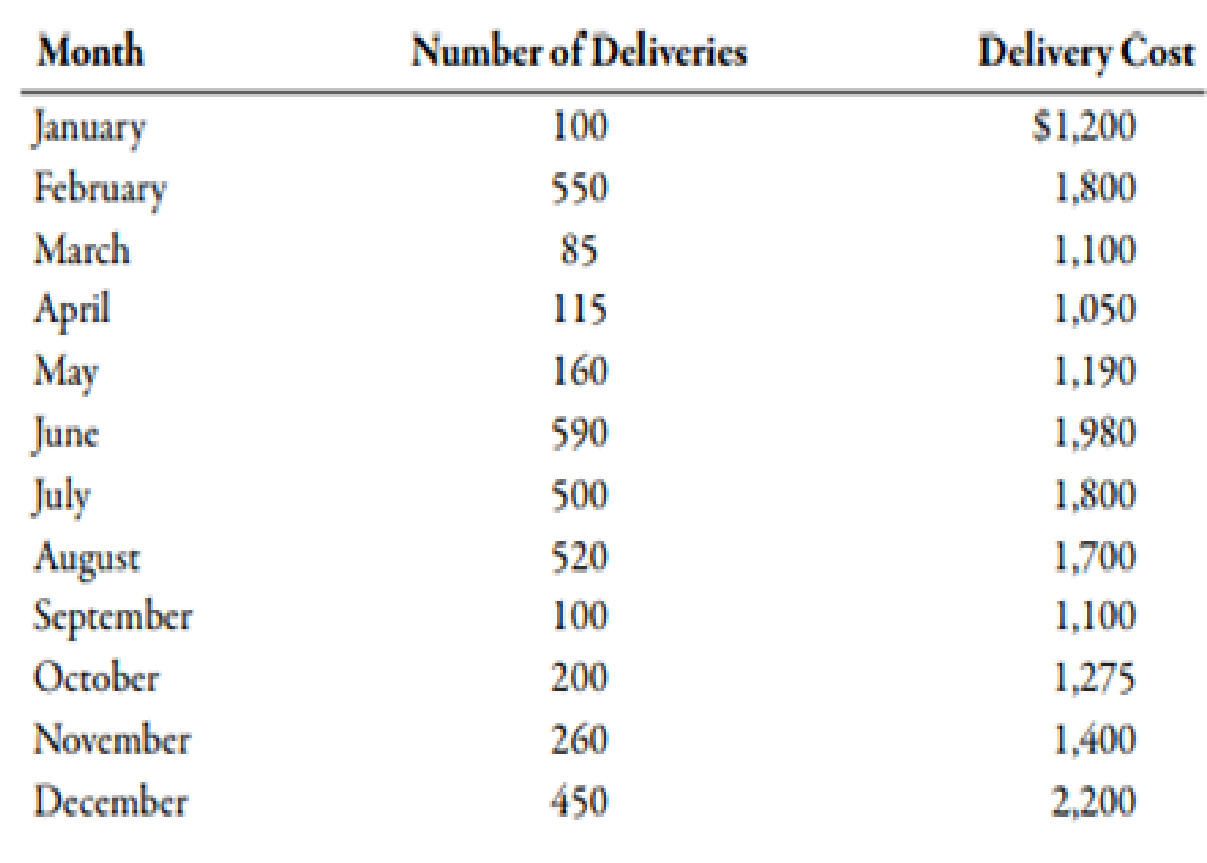Chapter 3, Problem 56E### Managerial Accounting: The Corners...

7th Edition
Maryanne M. Mowen + 2 others
ISBN: 9781337115773

#### Solutions

Chapter
Section### Managerial Accounting: The Corners...

7th Edition
Maryanne M. Mowen + 2 others
ISBN: 9781337115773
Textbook Problem
66 views

# (Appendix 3A) Method of Least Squares Using Computer Spreadsheet ProgramSusan Lewis, owner of a florist shop, is interested in predicting the cost of delivering floral arrangements. She collected monthly data on the number of deliveries and the total monthly delivery cost (depreciation on the van, wages of the driver, and fuel) for the past year.Required: 1. Using a computer spreadsheet program such as Excel, run a regression on these data. Print out your results. 2. Using your results from Requirement 1, write the cost formula for delivery cost. (Note: Round the fixed cost to the nearest dollar and the variable rate to the nearest cent.) 3. CONCEPTUAL CONNECTION What is R2 based on your results? Do you think that the number of direct labor hours is a good predictor of delivery cost? 4. Using the cost formula in Requirement 2, what would predicted delivery cost be for a month with 300 deliveries?

1.

To determine

Prepare a regression on excel sheet of the given data.

Explanation

Least Square Method:

Least square method is used to split mixed cost into fixed and variable components by using set of data points...

2.

To determine

Prepare the cost formula for overhead cost with the help of regression.

3.

To determine

Calculate the value of R2 with the help of excel. Also, identify whether the number of direct labor hours is a good predictor of factory overhead cost.

4.

To determine

Calculate the expected factory overhead cost with the help of cost formula.

### Still sussing out bartleby?

Check out a sample textbook solution.

See a sample solution

#### The Solution to Your Study Problems

Bartleby provides explanations to thousands of textbook problems written by our experts, many with advanced degrees!

Get Started